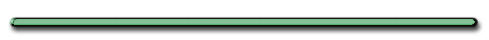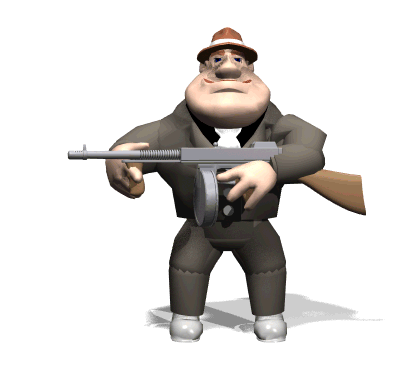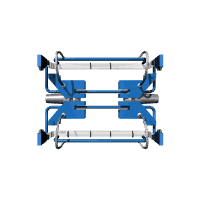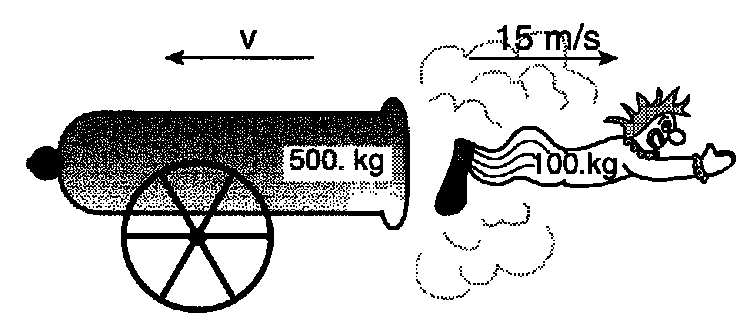Conservation of Momentum

Newton Collider

#23 - Recoil Problem - When interacting objects start from rest

 m1v1 = m2v2"What is the total momentum before cannon is fired?" "Total momentum after clown is fired?""Total momentum before=0. (at rest) "Total momentum after  = 0. (momenta are equal but opposite.)"

Rifle Recoil
Dr. Dave's Hall of Fame"In real life, it would be foolish to shoot a rifle  like this.  Why?""The recoil on that rifle would throw the shooter's shoulder out!"

 Ex 1) A 4.0 kg rifle fires a 5.0 x 10-3 kg bullet at a velocity of 500. m/s.  What is the velocity acquired by the rifle?(Not in Reference Table)Momenta before/after= 0 before

Equal Mass

W.F.U.

Total momentum after = 0 ??What is the velocity of the cannon below?m1v1 = m2v2

500 kg(V1) = 100 kg(15 m/s)

V1 = 3 m/s